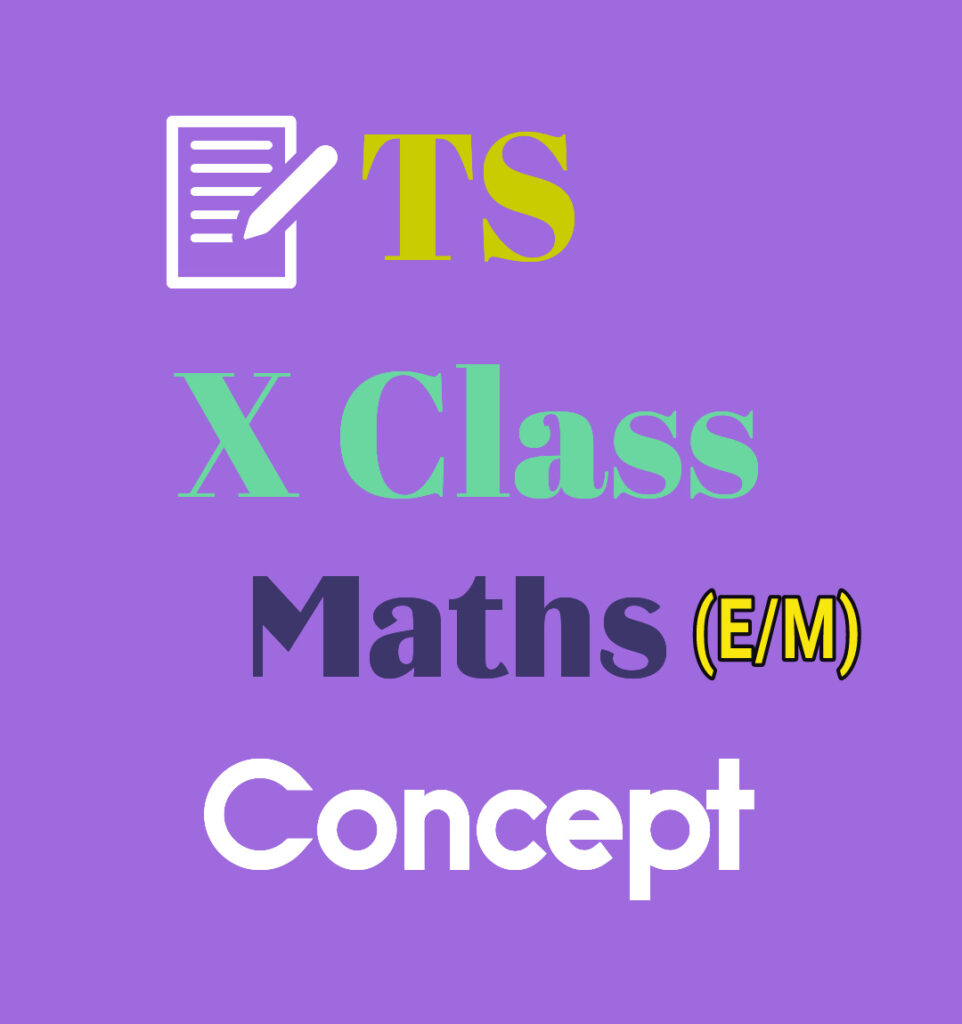Welcome To Basics In Maths

# TS 10th class maths concept (E/M)• Studying mathematics successfully meaning that, children take responsibility for their own learning and learn to apply the concepts to solve problems.

## 1. REAL NUMBERS

• Rational number: The number, which is written in the form of p/q where p,q are integers q not equal to zero is called a rational number. It is denoted by Q.

• Irrational numbers:- the number, which is not rational is called an irrational number. It is denoted by Q’ or S.

• Euclid division lemma:- For any positive integers a and b, then q, r are integers exists uniquely satisfying the rules a = bq + r, 0 ≤ r < b.

• Prime number:- The number which has only two factors 1 and itself is called a prime number. (2, 3, 5, 7 …. Etc.)

• Composite number:- the number which has more than two factors is called a composite number. (4, 6, 8, 9, 10,… etc.)

• Co-prime numbers:- Two numbers  said to be co-prime numbers if they have no common factor except 1. [Ex: (1, 2), (3, 4), (4, 7)…etc.]

• To find HCF, LCM by using prime factorization method:  H. C.F= product of the smallest power of each common prime factors of given numbers. L.C.M = product of the greatest power of each prime factor of given numbers.

• In p/q, if prime factorisation of q is in form 2m 5n, then p/q is terminating decimal. Otherwise non-terminating repeating decimal.
• Decimal numbers with the finite no. of digits is called terminating Decimal numbers with the infinite no. of digits is called non-terminating decimal. In a decimal, a digit or a sequence of digits in the decimal part keeps repeating itself infinitely. Such decimals are called non-terminating repeating decimals.

• ‘p’ is a prime number and ‘a’ is a positive integer, if p divides a2, then p divides a.

• If ax = N then x =

(i) log (xy) = log(x) + log(y)  (ii) log (x/y) = log( x) – log( y) (iii) log (xm ) = m log (x

Pair Of Linear Equations In two Variables Concept and Solutions: Click Here

Visit My Youtube Channel: Click on Below Logo